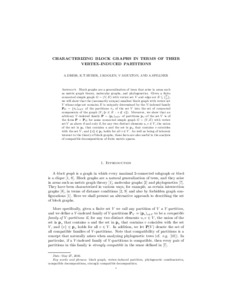# Characterizing block graphs in terms of their vertex-induced partitions

Dress, A, Huber, Katharina, Koolen, Jack, and Spillner, Andreas (2016) Characterizing block graphs in terms of their vertex-induced partitions. Australasian Journal of Combinatorics, 66 (1). pp. 1-9. ISSN 1034-4942Preview PDF (block-graph-final) - Accepted Version Download (178kB) | Preview

## Abstract

Block graphs are a generalization of trees that arise in areas such as metric graph theory, molecular graphs, and phylogenetics. Given a finite connected simple graph $G=(V,E)$ with vertex set $V$ and edge set $E\subseteq \binom{V}{2}$, we will show that the (necessarily unique) smallest block graph with vertex set $V$ whose edge set contains $E$ is uniquely determined by the $V$-indexed family $\Pp_G =\big(\pi_v)_{v \in V}$ of the partitions $\pi_v$ of the set $V$ into the set of connected components of the graph $(V,\{e\in E: v\notin e\})$. Moreover, we show that an arbitrary $V$-indexed family $\Pp=(\p_v)_{v \in V}$ of partitions $\p_v$ of the set $V$ is of the form $\Pp=\Pp_G$ for some connected simple graph $G=(V,E)$ with vertex set $V$ as above if and only if, for any two distinct elements $u,v\in V$, the union of the set in $\p_v$ that contains $u$ and the set in $\p_u$ that contains $v$ coincides with the set $V$, and $\{v\}\in \p_v$ holds for all $v \in V$. As well as being of inherent interest to the theory of block graphs,these facts are also useful in the analysis of compatible decompositions of finite metric spaces.

Item Type: Article block graph,vertex-induced partition,phylogenetic combinatorics, compatible decompositions,strongly compatible decomposition Faculty of Science > School of Computing Sciences Faculty of Science > Research Groups > Computational Biology > Phylogenetics (former - to 2018)Faculty of Science > Research Groups > Computational Biology > Computational biology of RNA (former - to 2018)Faculty of Science > Research Groups > Computational BiologyFaculty of Science > Research Groups > Norwich Epidemiology CentreFaculty of Medicine and Health Sciences > Research Groups > Norwich Epidemiology Centre Pure Connector 23 May 2016 15:01 14 Jun 2023 12:33 https://ueaeprints.uea.ac.uk/id/eprint/59003View Item